## 1 Introduction

Explainability has developed into a major issue in Artificial Intelligence, particularly in the context of sub-symbolic approaches based on Machine Learning . In contrast, results produced by symbolic approaches based on logical reasoning are “explainable by design” since a derived consequence can be formally justified by showing a proof for it. In practice, things are not that easy since proofs may be very long, and even single proof steps or stated sentences may be hard to comprehend for a user that is not an expert in logic. For this reason, there has been considerable work in the Automated Deduction and Logic in AI communities on how to produce “good” proofs for certain purposes, both for full first-order logic, but also for decidable logics such a Description Logics (DLs) . We mention here only a few approaches, and refer the reader to the introduction of our previous work  for a more detailed review.

First, there is work that transforms proofs that are produced by an automated reasoning system into ones in a calculus that is deemed to be more appropriate for human consumption [11, 22, 23]. Second, abstraction techniques are used to reduce the size of proofs by introducing definitions, lemmas, and more abstract deduction rules [16, 17]. Justification-based explanations for DLs [10, 14, 28] can be seen as a radical abstraction technique where the abstracted proof consists of a single proof step, from a minimal set of stated sentences that implies a certain consequence directly to this consequence. Finally, instead of presenting proofs in a formal, logical syntax, one can also try to increase readability by translating them into natural language text [12, 25,26,27] or visualizing them .

The purpose of this work is of a more (complexity) theoretic nature. We want to investigate how hard it is to find good proofs, where the quality of a proof is described by a measure $$\mathfrak {m}$$ that assigns non-negative rational numbers to proofs. More precisely, as usual we investigate the complexity of the corresponding decision problem, i.e., the problem of deciding whether there is a proof $$\mathcal {P}$$ with $$\mathfrak {m} (\mathcal {P})\le q$$ for a given rational number q. In order to abstract from specific logics and proof calculi, we develop a general framework in which proofs are represented as labeled, directed hypergraphs, whose hyperedges correspond to single sound derivation steps. To separate the complexity of generating good proofs from the complexity of reasoning in the underlying logic, we introduce the notion of a deriver, which generates a so-called derivation structure. This structure consists of possible proof steps, from which all proofs of the given consequence can be constructed. Basically, such a derivation structure can be seen as consisting of all relevant instantiations of the rules of a calculus that can be used to derive the consequence. We restrict the attention to decidable logics and consider derivers that produce derivation structures of polynomial or exponential size. Examples of such derivers are consequence-based reasoners for the DLs $$\mathcal {E}\mathcal {L}$$ [7, 21] and $$\mathcal {ELI}$$ [9, 18], respectively. In our complexity results, the derivation structure is assumed to be already computed by the deriver,Footnote 1 i.e., the complexity of this step is not assumed to be part of the complexity of computing good proofs. Our complexity results investigate the problem along the following orthogonal dimensions: we distinguish between (i) polynomial and exponential derivers; and (ii) whether the threshold value q is encoded in unary or binary. The obtained complexity upper bounds hold for all instances of a considered setting, whereas the lower bounds mean that there is an instance (usually based on $$\mathcal {E}\mathcal {L}$$ or $$\mathcal {ELI}$$) for which this lower bound can be proved.

In our first work in this direction , we focused our attention on size as the measure of proof quality. We could show that the above decision problem is NP-complete even for polynomial derivers and unary coding of numbers. For exponential derivers, the complexity depends on the coding of numbers: NP-complete (NExpTime-complete) for unary (binary) coding. For the related measure tree size (which assumes that the proof hypergraphs are tree-shaped, i.e. cannot reuse already derived consequences), the complexity turned out to be considerably lower, due to the fact that a Dijkstra-like greedy algorithm can be applied. In , we generalized the results by introducing a class of measures called $$\varPsi$$-measures, which contains both size and tree size and for which the same complexity upper bounds as for size could be shown for polynomial derivers. We also lifted the better upper bounds for tree size (for polynomial derivers) to local $$\varPsi$$-measures, a natural class of proof measures. In this paper, we extend this line of research by providing a more general notion of measures, monotone recursive $$\Phi$$-measures, which now also allow to measure the depth of a proof. We think that depth is an important measure since it measures how much of the proof tree a (human or automated) proof checker needs to keep in memory at the same time. We analyze these measures not only for polynomial derivers, but this time also consider exponential derivers, thus giving insights on how our complexity results transfer to more expressive logics. In addition to upper bounds for the general class of monotone recursive $$\mathrm {\Phi }$$-measures, we show improved bounds for the specific measures considering depth and tree size, in the latter case improving results from . Overall, we thus obtain a comprehensive picture of the complexity landscape for the problem of finding good proofs for DL and other entailments (see Table 1).

An extended version of this paper with detailed proofs can be found at .

## 2 Preliminaries

Most of our theoretical discussion applies to arbitrary logics $$\mathcal {L} =(\mathcal {S}_\mathcal {L},\models _\mathcal {L})$$ that consist of a set $$\mathcal {S}_\mathcal {L}$$ of $$\mathcal {L}$$-sentences and a consequence relation $${\models _\mathcal {L}}\subseteq P(\mathcal {S}_\mathcal {L})\times \mathcal {S}_\mathcal {L}$$ between $$\mathcal {L}$$ -theories, i.e. subsets of $$\mathcal {L}$$-sentences, and single $$\mathcal {L}$$-sentences. We assume that $$\models _\mathcal {L}$$ has a semantic definition, i.e. for some definition of “model”, $$\mathcal {T} \models _\mathcal {L} \eta$$ holds iff every model of all elements in $$\mathcal {T}$$ is also a model of $$\eta$$. We also assume that the size $$|\eta |$$ of an $$\mathcal {L}$$-sentence $$\eta$$ is defined in some way, e.g. by the number of symbols in $$\eta$$. Since $$\mathcal {L}$$ is usually fixed, we drop the prefix “$$\mathcal {L}$$-” from now on. For example, $$\mathcal {L}$$ could be first-order logic. However, we are mainly interested in proofs for DLs, which can be seen as decidable fragments of first-order logic . In particular, we use specific DLs to show our hardness results.

The syntax of DLs is based on disjoint, countably infinite sets $$\textsf {N}_\textsf {C}$$ and $$\textsf {N}_\textsf {R}$$ of concept names $$A,B,\dots$$ and role names $$r,s,\dots$$, respectively. Sentences of the DL $$\mathcal {E}\mathcal {L}$$, called general concept inclusions (GCIs), are of the form $$C\sqsubseteq D$$, where C and D are $$\mathcal {E}\mathcal {L}$$ -concepts, which are built from concept names by applying the constructors $$\top$$ (top), $$C\sqcap D$$ (conjunction), and $$\exists r.C$$ (existential restriction for a role name r). The DL $$\mathcal {ELI}$$ extends $$\mathcal {E}\mathcal {L}$$ by the role constructor $$r^-$$ (inverse role). In DLs, finite theories are called TBoxes or ontologies.

The semantics of DLs is based on first-order interpretations; for details, see . In Figure 1, we depict a simplified version of the inference rules for $$\mathcal {E}\mathcal {L}$$ from . For example, $$\{A\sqsubseteq \exists r.B,\ B\sqsubseteq C,\ \exists r.C\sqsubseteq D\}\models A\sqsubseteq D$$ is a valid inference in $$\mathcal {E}\mathcal {L}$$. Deciding consequences in $$\mathcal {E}\mathcal {L}$$ is P-complete , and in $$\mathcal {ELI}$$ it is ExpTime-complete .

### 2.1 Proofs

We formalize proofs as (labeled, directed) hypergraphs (see Figures 23), which are tuples $$(V,E,\ell )$$ consisting of a finite set V of vertices, a finite set E of (hyper)edges of the form (Sd) with $$S\subseteq V$$ and $$d\in V$$, and a vertex labeling function $$\ell :V\rightarrow \mathcal {S}_\mathcal {L}$$. Full definitions of such hypergraphs, as well as related notions such as trees, unravelings, homomorphisms, cycles can be found in the extended version . For example, there is a homomorphism from Figure 3 to Figure 2, but not vice versa, and Figure 3 is the tree unraveling of Figure 2.

The following definition formalizes basic requirements for hyperedges to be considered valid inference steps from a given finite theory.

### Definition 1

(Derivation Structure). A derivation structure $$\mathcal {D} = (V, E, \ell )$$ over a finite theory $$\mathcal {T}$$ is a hypergraph that is

• grounded, i.e. every leaf v in $$\mathcal {D}$$ is labeled by $$\ell (v)\in \mathcal {T}$$; and

• sound, i.e. for every $$(S,d)\in E$$, the entailment $$\{\ell (s)\mid s\in S\}\models \ell (d)$$ holds.

We define proofs as special derivation structures that derive a conclusion.

### Definition 2

(Proof). Given a conclusion $$\eta$$ and a finite theory $$\mathcal {T}$$, a proof for $$\mathcal {T} \models \eta$$ is a derivation structure $$\mathcal {P} = (V, E,\ell )$$ over $$\mathcal {T}$$ such that

• $$\mathcal {P}$$ contains exactly one sink $$v_\eta \in V$$, which is labeled by $$\eta$$,

• $$\mathcal {P}$$ is acyclic, and

• every vertex has at most one incoming edge, i.e. there is no vertex $$w\in V$$ s.t. there are $$(S_1,w), (S_2,w)\in E$$ with $$S_1\ne S_2$$.

A tree proof is a proof that is a tree. A subproof S of a hypergraph H is a subgraph of H that is a proof s.t. the leaves of S are a subset of the leaves of H.

The hypergraphs in Figures 2 and 3 can be seen as proofs in the sense of Definition 2, where the sentences of the theory are marked with a thick border. Both proofs use the same inference steps, but have different numbers of vertices. They both prove $$A\sqsubseteq B\sqcap \exists r.A$$ from $$\mathcal {T} =\{ A \sqsubseteq B,\ B \sqsubseteq \exists r.A \}$$. The second proof is a tree and the first one a hypergraph without label repetition.

### Lemma 3

Let $$\mathcal {P} =(V,E,\ell )$$ be a proof for $$\mathcal {T} \models \eta$$. Then

1. 1.

all paths in $$\mathcal {P}$$ are finite and all longest paths in $$\mathcal {P}$$ have $$v_\eta$$ as the target; and

2. 2.

$$\mathcal {T} \models \eta$$.

Given a proof $$\mathcal {P} =(V, E,\ell )$$ and a vertex $$v\in V$$, the subproof of $$\mathcal {P}$$ with sink v is the largest subgraph $$\mathcal {P} _v=(V_v,E_v,\ell _v)$$ of $$\mathcal {P}$$ where $$V_v$$ contains all vertices in V that have a path to v in $$\mathcal {P}$$.

### 2.2 Derivers

In practice, proofs and derivation structures are constructed by a reasoning system, and in theoretical investigations, it is common to define proofs by means of a calculus. To abstract from these details, we use the concept of a deriver as in , which is a function that, given a theory $$\mathcal {T}$$ and a conclusion $$\eta$$, produces the corresponding derivation structure in which we can look for an optimal proof. However, in practice, it would be inefficient and unnecessary to compute the entire derivation structure beforehand when looking for an optimal proof. Instead, we allow to access elements in a derivation structure using an oracle, which we can ask whether given inferences are a part of the current derivation structure. Similar functionality exists for example for the DL reasoner Elk , and may correspond to checking whether the inference is an instance of a rule in the calculus. Since reasoners may not be complete for proving arbitrary sentences of $$\mathcal {L}$$, we restrict the conclusion $$\eta$$ to a subset $$C_\mathcal {L} \subseteq \mathcal {S}_\mathcal {L}$$ of supported consequences.

### Definition 4

(Deriver). A deriver $$\mathfrak {D}$$ is given by a set $$C_\mathcal {L} \subseteq \mathcal {S}_\mathcal {L}$$ and a function that assigns derivation structures to pairs $$(\mathcal {T},\eta )$$ of finite theories $$\mathcal {T} \subseteq \mathcal {S}_\mathcal {L}$$ and sentences $$\eta \in C_\mathcal {L}$$, such that $$\mathcal {T} \models \eta$$ iff $$\mathfrak {D} (\mathcal {T},\eta )$$ contains a proof for $$\mathcal {T} \models \eta$$. A proof $$\mathcal {P}$$ for $$\mathcal {T} \models \eta$$ is called admissible w.r.t. $$\mathfrak {D} (\mathcal {T},\eta )$$ if there is a homomorphism $$h:\mathcal {P} \rightarrow \mathfrak {D} (\mathcal {T},\eta )$$. We call $$\mathfrak {D}$$ a polynomial deriver if there exists a polynomial p(x) such that the size of $$\mathfrak {D} (\mathcal {T},\eta )$$ is bounded by $$p(|\mathcal {T} |+|\eta |)$$. Exponential deriver s are defined similarly by the restriction $$|\mathfrak {D} (\mathcal {T},\eta )|\le 2^{p(|\mathcal {T} |+|\eta |)}$$.

Elk is an example of a polynomial deriver, that is, for a given $$\mathcal {E}\mathcal {L}$$  theory $$\mathcal {T}$$ and $$\mathcal {E}\mathcal {L}$$ sentence $$\eta$$,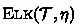contains all allowed instances of the rules shown in Figure 1. As an example for an exponential deriver we use Eli, which uses the rules from Figure 4 and is complete for $$\mathcal {ELI}$$ theories and conclusions of the form $$A\sqsubseteq B$$, A, $$B\in \textsf {N}_\textsf {C}$$. The oracle access for a deriver $$\mathfrak {D}$$ works as follows. Let $$\mathcal {D} =(V,E,\ell ):=\mathfrak {D} (\mathcal {T},\eta )$$ and $$V=\{v_1,\dots ,v_m\}$$. $$\mathcal {D}$$ is accessed using the following two functions, where $$i,i_1,\dots ,i_l$$ are indices of vertices and $$\alpha$$ is a sentence:

\begin{aligned}{}[\mathcal {D} ](i_1,\dots ,i_l,i)&:= {\left\{ \begin{array}{ll} \mathsf {true} &{}\text {if }(\{v_{i_1},\dots ,v_{i_{l}}\},v_i)\in E, \\ \mathsf {false} &{}\text {otherwise;} \end{array}\right. } \\ [\mathcal {D} ](i,\alpha )&:= {\left\{ \begin{array}{ll} \mathsf {true} &{} \text {if } \ell (v_i)=\alpha , \\ \mathsf {false} &{} \text {otherwise.} \end{array}\right. } \end{aligned}

In this paper, we focus on polynomial and exponential derivers, for which we further make the following technical assumptions: 1) $$\mathfrak {D} (\mathcal {T},\eta )$$ does not contain two vertices with the same label; 2) the number of premises in an inference is polynomially bounded by $$|\mathcal {T} |$$ and $$|\eta |$$; and 3) the size of each label is polynomially bounded by $$|\mathcal {T} |$$ and $$|\eta |$$. While 1) is without loss of generality, 2) and 3) are not. If a deriver does not satisfy 2), we may be able to fix this by splitting inference steps. Assumption 3) would not work for derivers with higher complexity, but is required in our setting to avoid trivial complexity results for exponential derivers. We furthermore assume that for polynomial and exponential derivers, the polynomial p from Definition 4 bounding the size of derivation structures is known.

## 3 Measuring Proofs

To formally study quality measures for proofs, we developed the following definition, which will be instantiated with concrete measures later. Our goal is to find proofs that minimize these measures, i.e. lower numbers are better.

### Definition 5

($$\boldsymbol{\Phi }$$-Measure). A (quality) measure is a function $$\mathfrak {m} :\mathrm {P}_{\mathcal {L}} \rightarrow \mathbb {Q} _{\ge 0}$$, where $$\mathrm {P}_{\mathcal {L}}$$ is the set of all proofs over $$\mathcal {L}$$ and $$\mathbb {Q} _{\ge 0}$$ is the set of non-negative rational numbers. We call $$\mathfrak {m}$$ a $$\mathrm {\Phi }$$ -measure if, for every $$\mathcal {P} \in \mathrm {P}_{\mathcal {L}}$$, the following hold.

[P]:

$$\mathfrak {m} (\mathcal {P})$$ is computable in polynomial time in the size of $$\mathcal {P}$$.

[HI]:

Let $$h:\mathcal {P} \rightarrow H$$ be any homomorphism, and $$\mathcal {P} '$$ be any subproof of the homomorphic image $$h(\mathcal {P})$$ that is minimal (w.r.t. $$\mathfrak {m}$$) among all such subproofs having the same sink. Then $$\mathfrak {m} (\mathcal {P} ')\le \mathfrak {m} (\mathcal {P})$$.

Intuitively, a $$\mathrm {\Phi }$$-measure $$\mathfrak {m}$$ does not increase when the proof gets smaller, either when parts of the proof are removed (to obtain a subproof) or when parts are merged (in a homomorphic image). For example, $$\mathfrak {m} _{\mathsf {size}} ((V,E,\ell )):=|V|$$ is a $$\mathrm {\Phi }$$-measure, called the size of a proof, and we have already investigated the complexity of the following deicision problem for $$\mathfrak {m} _{\mathsf {size}}$$ in .

### Definition 6

(Optimal Proof). Let $$\mathfrak {D}$$ be a deriver and $$\mathfrak {m}$$ be a measure. Given a finite theory $$\mathcal {T}$$ and a sentence $$\eta \in C_\mathcal {L}$$ s.t. $$\mathcal {T} \models \eta$$, an admissible proof $$\mathcal {P}$$ w.r.t. $$\mathfrak {D} (\mathcal {T},\eta )$$ is called optimal w.r.t. $$\mathfrak {m}$$ if $$\mathfrak {m} (\mathcal {P})$$ is minimal among all such proofs. The associated decision problem, denoted $$\mathsf {OP} (\mathfrak {D},\mathfrak {m})$$, is to decide, given $$\mathcal {T}$$ and $$\eta$$ as above and $$q\in \mathbb {Q} _{\ge 0}$$, whether there is an admissible proof $$\mathcal {P}$$ w.r.t. $$\mathfrak {D} (\mathcal {T},\eta )$$ with $$\mathfrak {m} (\mathcal {P})\le q$$.

For our complexity analysis, we distinguish the encoding of q with a subscript ($$\mathsf {unary}$$/$$\mathsf {binary}$$), e.g. $$\mathsf {OP} _\mathsf {unary} (\mathfrak {D},\mathfrak {m})$$.

We first show that if $$\mathcal {P}$$ is optimal w.r.t. a $$\mathrm {\Phi }$$-measure $$\mathfrak {m}$$ and $$\mathfrak {D} (\mathcal {T},\eta )$$, then the homomorphic image of $$\mathcal {P}$$ in $$\mathfrak {D} (\mathcal {T},\eta )$$ is also a proof. Thus, to decide $$\mathsf {OP} (\mathfrak {D},\mathfrak {m})$$ we can restrict our search to proofs that are subgraphs of $$\mathfrak {D} (\mathcal {T},\eta )$$.

### Lemma 7

For any deriver $$\mathfrak {D}$$ and $$\mathrm {\Phi }$$-measure $$\mathfrak {m}$$, if there is an admissible proof $$\mathcal {P}$$ w.r.t. $$\mathfrak {D} (\mathcal {T},\eta )$$ with $$\mathfrak {m} (\mathcal {P})\le q$$ for some $$q\in \mathbb {Q} _{\ge 0}$$, then there exists a subproof $$\mathcal {Q}$$ of $$\mathfrak {D} (\mathcal {T},\eta )$$ for $$\mathcal {T} \models \eta$$ with $$\mathfrak {m} (\mathcal {Q})\le q$$.

In particular, this shows that an optimal proof always exists.

### Corollary 8

For any deriver  $$\mathfrak {D}$$ and $$\mathrm {\Phi }$$-measure $$\mathfrak {m}$$, if $$\mathcal {T} \models \eta$$, then there is an optimal proof for $$\mathcal {T} \models \eta$$ w.r.t. $$\mathfrak {D}$$ and $$\mathfrak {m}$$.

### Proof

By Definition 4, the derivation structure $$\mathfrak {D} (\mathcal {T},\eta )$$ contains at least one proof for $$\mathcal {T} \models \eta$$. Since $$\mathfrak {D} (\mathcal {T},\eta )$$ is finite, there are finitely many proofs for $$\mathcal {T} \models \eta$$ contained in $$\mathfrak {D} (\mathcal {T},\eta )$$. The finite set of all $$\mathfrak {m}$$-weights of these proofs always has a minimum. Finally, if there were an admissible proof weighing less than this minimum, it would contradict Lemma 7.    $$\square$$

### 3.1 Monotone Recursive Measures

Since the complexity of $$\mathsf {OP} (\mathfrak {D},\mathfrak {m})$$ for $$\mathrm {\Phi }$$-measures in general is quite high , in this paper we focus on a subclass of measures that can be evaluated recursively.

### Definition 9

A $$\mathrm {\Phi }$$-measure $$\mathfrak {m}$$ is recursive if there exist

• a leaf function $$\mathsf {leaf} _\mathfrak {m} :\mathcal {S}_\mathcal {L} \rightarrow \mathbb {Q}_{\ge 0}$$ and

• a partial edge function $$\mathsf {edge} _\mathfrak {m}$$, which maps (i) the labels $$(\mathcal {S},\alpha )$$ of a hyperedge and (ii) a finite multiset $$\mathcal {Q}$$ of already computed intermediate weights in $$\mathbb {Q}_{\ge 0}$$ to a combined weight $$\mathsf {edge} _\mathfrak {m} \big ((\mathcal {S},\alpha ),\mathcal {Q} \big )$$

such that, for any proof $$\mathcal {P} =(V,E,\ell )$$ with sink v, we have

$$\mathfrak {m} (\mathcal {P})={\left\{ \begin{array}{ll} \mathsf {leaf} _\mathfrak {m} (\ell (v)) &{} \text {if } V=\{v\},\\ \mathsf {edge} _\mathfrak {m} \big (\ell (S,v),\{\mathfrak {m} (\mathcal {P} _w)\mid w\in S\}\big ) &{} \text {if }(S,v)\in E. \end{array}\right. }$$

Such a measure is monotone if, for any multiset $$\mathcal {Q}$$, whenever $$q\in \mathcal {Q}$$ and $$\mathcal {Q} '=(\mathcal {Q} \setminus \{q\})\cup \{q'\}$$ with $$q'\le q$$ and both $$\mathsf {edge} _\mathfrak {m} \big ((\mathcal {S},\alpha ),\mathcal {Q} '\big )$$ and $$\mathsf {edge} _\mathfrak {m} \big ((\mathcal {S},\alpha ),\mathcal {Q} \big )$$ are defined, then $$\mathsf {edge} _\mathfrak {m} \big ((\mathcal {S},\alpha ),\mathcal {Q} '\big )\le \mathsf {edge} _\mathfrak {m} \big ((\mathcal {S},\alpha ),\mathcal {Q} \big )$$.

Intuitively, a recursive measure $$\mathfrak {m}$$ can be computed in a bottom-up fashion starting with the weights of the leaves given by $$\mathsf {leaf} _\mathfrak {m}$$. The function $$\mathsf {edge} _\mathfrak {m}$$ is used to recursively combine the weights of the direct subproofs into a weight for the full proof. This function is well-defined since in a proof every vertex has at most one incoming edge. We require $$\mathsf {edge} _\mathfrak {m}$$ to be defined only for inputs $$\big ((\mathcal {S},\alpha ),\mathcal {Q} \big )$$ that actually correspond to a valid proof in $$\mathcal {L}$$, i.e. where $$\mathcal {S} \models _\mathcal {L} \alpha$$ and $$\mathcal {Q}$$ consists of the weights of some proofs for the sentences in $$\mathcal {S}$$. For example, if $$\mathfrak {m}$$ always yields natural numbers, we obviously do not need $$\mathsf {edge} _\mathfrak {m}$$ to be defined for multisets containing fractional numbers.

In this paper, we are particularly interested in the following monotone recursive $$\mathrm {\Phi }$$-measures.

• The depth $$\mathfrak {m} _{\mathsf {depth}}$$ of a proof is defined by

$$\mathsf {leaf} _{\mathfrak {m} _{\mathsf {depth}}}(\alpha ):=0 \text { and } \mathsf {edge} _{\mathfrak {m} _{\mathsf {depth}}}\big ((\mathcal {S},\alpha ),\mathcal {Q} \big ):=1+\max \mathcal {Q}.$$
• The tree size $$\mathfrak {m} _{\mathsf {tree}}$$ is given by

$$\mathsf {leaf} _{\mathfrak {m} _{\mathsf {tree}}}(\alpha ):=1 \text { and } \mathsf {edge} _{\mathfrak {m} _{\mathsf {tree}}}\big ((\mathcal {S},\alpha ),\mathcal {Q} \big ):=1+\sum \mathcal {Q}.$$

What distinguishes tree size from size is that vertices are counted multiple times if they are used in several subproofs. The name tree size is inspired by the fact that it can be interpreted as the size of the tree unraveling of a given proof (cf. Figures 2 and 3). In fact, we show in the extended version  that all recursive $$\mathrm {\Phi }$$-measures are invariant under unraveling. This indicates that tree size, depth and other monotone recursive $$\mathrm {\Phi }$$-measures are especially well-suited for cases where proofs are presented to users in the form of trees. This is for example the case for the proof plugin for Protégé  .

### Lemma 10

Depth and tree size are monotone recursive $$\mathrm {\Phi }$$-measures.

## 4 Complexity Results

We investigate the decision problem $$\mathsf {OP}$$ for monotone recursive $$\mathrm {\Phi }$$-measures. We first show upper bounds for the general case, and then consider measures for depth and tree size, for which we obtain even lower bounds. An artificial modification of the depth measure gives a lower bound matching the general upper bound even if unary encoding is used for the threshold q.

### 4.1 The General Case

Algorithm 1 describes a Dijkstra-like approach that is inspired by the algorithm in  for finding minimal hyperpaths w.r.t. so-called additive weighting functions, which represent a subclass of monotone recursive $$\mathrm {\Phi }$$-measures. The algorithm progressively discovers proofs $$\mathcal {P} (v)$$ for $$\ell (v)$$ that are contained in $$\mathfrak {D} (\mathcal {T},\eta )$$. If it reaches a new vertex v in this process, this vertex is added to the set Q. In each step, a vertex with minimal weight $$\mathfrak {m} (\mathcal {P} (v))$$ is chosen and removed from Q. For each hyperedge $$e=(S,d)\in E$$, a counter k(e) is maintained that is increased whenever a vertex $$v\in S$$ is chosen. Once this counter reaches |S|, we know that all source vertices of e have been processed. The algorithm then constructs a new proof $$\mathcal {P}$$ for $$\ell (d)$$ by joining the proofs for the source vertices using the current hyperedge e. This proof $$\mathcal {P}$$ is then compared to the best previously known proof $$\mathcal {P} (d)$$ for $$\ell (d)$$ and $$\mathcal {P} (d)$$ is updated accordingly. For Line 20, recall that we assumed $$\mathfrak {D} (\mathcal {T},\eta )$$ to contain no two vertices with the same label, and hence it contains a unique vertex $$v_\eta$$ with label $$\eta$$.

### Lemma 11

For any monotone recursive $$\mathrm {\Phi }$$-measure $$\mathfrak {m}$$ and deriver  $$\mathfrak {D}$$, Algorithm 1 computes an optimal proof in time polynomial in the size of $$\mathfrak {D} (\mathcal {T},\eta )$$.

Since we can actually compute an optimal proof in polynomial time in the size of the whole derivation structure, it is irrelevant how the upper bound q in the decision problem $$\mathsf {OP}$$ is encoded, and hence the following results follow.

### Theorem 12

For any monotone recursive $$\mathrm {\Phi }$$-measure $$\mathfrak {m}$$ and polynomial deriver $$\mathfrak {D}$$, $$\mathsf {OP} _\mathsf {binary} (\mathfrak {D},\mathfrak {m})$$ is in P. It is in ExpTime for all exponential derivers $$\mathfrak {D}$$.

### 4.2 Proof Depth

We now consider the measure $$\mathfrak {m} _{\mathsf {depth}}$$ in more detail. We can show lower bounds of P and ExpTime for polynomial and exponential derivers, respectively, although the latter only holds for upper bounds q encoded in binary.

Since our definition of $$\mathsf {OP} (\mathfrak {D},\mathfrak {m})$$ requires that the input entailment $$\mathcal {T} \models \eta$$ already holds, we cannot use a straightforward reduction from the entailment problem in $$\mathcal {E}\mathcal {L}$$ or $$\mathcal {ELI}$$, however. Instead, we show that ordinary proofs $$\mathcal {P}$$ for $$\mathcal {T} \models \eta$$ satisfy $$\mathfrak {m} (\mathcal {P})\le q$$ for some q, and then extend the TBox to $$\mathcal {T} '$$ in order to create an artificial proof $$\mathcal {P} '$$ with $$\mathfrak {m} (\mathcal {P} ')>q$$. In this way, we ensure that $$\mathcal {T} '\models \eta$$ holds and can use q to distinguish the artificial from the original proofs.

For $$\mathcal {ELI}$$, we can use an observation from [9, Example 6.29] for this purpose.

### Proposition 13

(). For every $$q\in \mathbb {Q}_{\ge 0}$$ and $$\mathcal {ELI}$$ sentence of the form $$A\sqsubseteq B$$, where $$A,B\in \textsf {N}_\textsf {C}$$, one can construct in time polynomial in q an $$\mathcal {ELI}$$ theory $$\mathcal {T}$$ such $$\mathcal {T} \models A\sqsubseteq B$$, and every proof for $$\mathcal {T} \models A\sqsubseteq B$$ in Eli is of depth larger than $$2^q$$.

We can now reduce the entailment problems for $$\mathcal {E}\mathcal {L}$$ and $$\mathcal {ELI}$$ to obtain the claimed lower bounds.

### Theorem 14

The problems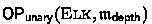and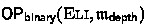are P-hard and ExpTime-hard, respectively.

### Proof

For the P-hardness, we provide a LogSpace-reduction from the entailment problem of a GCI $$A\sqsubseteq B$$ with two concept names AB from an $$\mathcal {E}\mathcal {L}$$-theory $$\mathcal {T}$$, which is P-hard . To reduce this problem to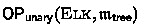, we need to find a theory $$\mathcal {T} '$$ and a number q such that $$\mathcal {T} '\models A\sqsubseteq B$$ holds, and moreover $$\mathcal {T} \models A\sqsubseteq B$$ holds iff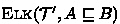contains a proof of $$\mathcal {T} '\models A\sqsubseteq B$$ of depth $$\le q$$ (cf. Lemma 7).

First, observe that, since proofs must be acyclic, the depth of any proof of $$A\sqsubseteq B$$ from $$\mathcal {T}$$ is bounded by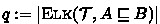, whose size in unary encoding is polynomial in the size of $$\mathcal {T}$$. We now construct

\begin{aligned} \mathcal {T} ' := \mathcal {T} \cup \{ A\sqsubseteq A_1,\ A_1\sqsubseteq A_2, \dots , A_{q+2}\sqsubseteq B\}, \end{aligned}

where $$A_1,\dots ,A_q$$ are concept names not occurring in $$\mathcal {T}$$. Clearly, we have $$\mathcal {T} '\models A\sqsubseteq B$$. Furthermore, the existence of an admissible proof for $$\mathcal {T} '\models A\sqsubseteq B$$ of depth at most q is equivalent to $$\mathcal {T} \models A\sqsubseteq B$$, since any proof that uses the new concept names must take $$q+1$$ consecutive steps using rule $$\mathsf {R}_\sqsubseteq$$, i.e. must be of depth $$q+1$$. Moreover, we can compute q (in binary representation) and output it in unary representation using a logarithmically space-bounded Turing machine, and similarly for $$\mathcal {T} '$$. Hence, the above construction constitutes the desired LogSpace-reduction.

For the remaining result, we can use similar arguments about the exponential deriver Eli, where entailment is ExpTime-hard :

• the minimal depth of a proof in an exponential derivation structure is at most exponential, and this exponential bound q can be computed in polynomial time using binary encoding;

• by Proposition 13, there is an $$\mathcal {ELI}$$ theory $$\mathcal {T}$$ of size polynomial in the size of the binary encoding of q such that $$\mathcal {T} \models A\sqsubseteq B$$ and any proof for $$\mathcal {T} \models A\sqsubseteq B$$ must have at least depth $$q+1$$.    $$\square$$

To demonstrate that the generic upper bounds from Theorem 12 are tight even for unary encoding, we quickly consider the artificial measure $$\mathfrak {m} _{\mathsf {log(depth)}}$$ (logarithmic depth), which simply computes the (binary) logarithm of the depth of a given proof. This is also a monotone recursive $$\mathrm {\Phi }$$-measure, since the logarithmic depth contains exactly the same information as the depth itself. It is easy to obtain the following lower bounds from the previous results about $$\mathfrak {m} _{\mathsf {depth}}$$.

### Corollary 15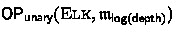is P-hard and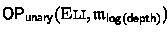is ExpTime-hard.

### Proof

For any deriver $$\mathfrak {D}$$, $$\mathsf {OP} _\mathsf {binary} (\mathfrak {D},\mathfrak {m} _{\mathsf {depth}})$$ can be LogSpace-reduced to $$\mathsf {OP} _\mathsf {unary} (\mathfrak {D},\mathfrak {m} _{\mathsf {log(depth)}})$$, because in order to find a proof of depth at most q (with q given in binary), one can equivalently look for a proof whose logarithmic depth is bounded by the value $$\log q$$. The unary encoding of $$\log q$$ has the same size as the binary encoding of q and can be computed in LogSpace by flipping all bits of the binary encoding of q to 1.    $$\square$$

We now return to $$\mathfrak {m} _{\mathsf {depth}}$$ and cover the remaining case of exponential derivers and unary encoding of the upper bound q.

### Theorem 16

$$\mathsf {OP} _\mathsf {unary} (\mathfrak {D},\mathfrak {m} _{\mathsf {depth}})$$ is in PSpace for any exponential deriver $$\mathfrak {D}$$. It is PSpace-hard for the exponential deriver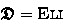.

### Proof

For the upper bound, we employ a depth-first guessing strategy: we guess a proof of depth at most q, where at each time point we only keep one branch of the proof in memory. As the length of this branch is bounded by q, and due to our assumptions on derivers, this procedure only requires polynomial space.

For the lower bound, we provide a reduction from the PSpace-complete QBF problem (satisfiability of quantified Boolean formulas). Let $${\mathsf {Q}}_1 x_1{\mathsf {Q}}_2 x_2\ldots {\mathsf {Q}}_m x_m.\phi$$ be a quantified Boolean formula, where for $$i\in \{1,\ldots ,m\}$$, $${\mathsf {Q}}_i\in \{{\exists },{\forall }\}$$, and $$\phi$$ is a formula over $$\{x_1,\ldots ,x_m\}$$. We assume $$\phi$$ to be in negation normal form, that is, negation only occurs directly in front of a variable. We construct an $$\mathcal {ELI}$$ theory $$\mathcal {T}$$ and a number q, both of size polynomial in the size of the formula, such that $$\mathcal {T} \models A\sqsubseteq B$$ holds (cf. Definition 6) and $$\mathcal {T}$$ has a proof for $$A\sqsubseteq B$$ of depth q iff the QBF formula is valid. We use two roles $$r_1$$, $$r_2$$ to deal with the variable valuations, concept names $$A_0$$, $$\ldots$$, $$A_{m}$$ to count the quantifier nesting, and a concept name $$A_\psi$$ for every sub-formula $$\psi$$ of $$\phi$$. In addition, we use the concept names A and B occurring in the conclusion, and two concept names $$B_1$$ and $$B_2$$.

The concept name A initializes the formula at quantifier nesting level 0:

\begin{aligned} A\sqsubseteq A_0 \end{aligned}

For every $$i\in \{1,\ldots ,m\}$$, $$\mathcal {T}$$ contains the following sentence to select a truth valuation for $$x_i$$, increasing the nesting depth in each step.

\begin{aligned} A_{i-1}&\sqsubseteq \exists r_1.(A_{i}\sqcap A_{x_i})\end{aligned}
(1)
\begin{aligned} A_{i-1}&\sqsubseteq \exists r_2.(A_{i}\sqcap A_{\lnot x_i}). \end{aligned}
(2)

To ensure truth valuations are kept along the role-successors, we use the following sentences for every $$l\in \{x_i,\lnot x_i\mid 1\le i\le m\}$$:

\begin{aligned} A_l&\sqsubseteq \forall r_1.A_l \qquad A_l\sqsubseteq \forall r_2.A_l \end{aligned}
(3)

The following GCIs are now used to evaluate $$\phi$$. For every conjunction $$\psi =\psi _1\wedge \psi _2$$ occurring in $$\phi$$, we use:

\begin{aligned} A_{\psi _1}\sqcap A_{\psi _2}\sqsubseteq A_\psi , \end{aligned}
(4)

and for every disjunction $$\psi =\psi _1\vee \psi _2$$, we use:

\begin{aligned} A_{\psi _1}\sqsubseteq A_\psi \qquad A_{\psi _2}\sqsubseteq A_\psi \end{aligned}
(5)

Finally, the following GCIs are used to propagate the result of the evaluation back towards the start.

\begin{aligned} A_\phi&\sqsubseteq B \end{aligned}
(6)
\begin{aligned} A_i\sqcap B&\sqsubseteq \forall r_1^-.B\quad&A_i\sqcap B&\sqsubseteq \forall r_2^-.B&\quad \text { if }{\mathsf {Q}}_i={\exists }\end{aligned}
(7)
\begin{aligned} A_i\sqcap B&\sqsubseteq \forall r_1^-.B_1\qquad&A_i\sqcap B&\sqsubseteq \forall r_2^-.B_2 \qquad B_1\sqcap B_2\sqsubseteq B&\quad \text { if }{\mathsf {Q}}_i={\forall } \end{aligned}
(8)

One can now show that there exists a proof for $$A\sqsubseteq B$$ from $$\mathcal {T}$$ of depth at most q iff the QBF formula is valid, where q is polynomial and determined by the size and structure of $$\phi$$. Finally, we can extend $$\mathcal {T}$$ with the sentences from Proposition 13 to ensure that $$\mathcal {T} \models A\sqsubseteq B$$ holds while retaining this equivalence.    $$\square$$

### 4.3 The Tree Size Measure

The tree size measure was discussed already in , where tight bounds were provided for polynomial derivers and exponential derivers with unary encoding. For the case of exponential derivers with binary encoding, only an ExpTime upper bound was provided, and the precise complexity left open. We improve this result by showing that $$\mathsf {OP} _\mathsf {binary} (\mathfrak {D},\mathfrak {m} _{\mathsf {tree}})$$ can indeed be decided in PSpace.

### Theorem 17

For any exponential deriver $$\mathfrak {D}$$, $$\mathsf {OP} _\mathsf {binary} (\mathfrak {D},\mathfrak {m} _{\mathsf {tree}})$$ is in PSpace.

### Proof (sketch)

We describe a non-deterministic procedure for $$\mathsf {OP} _\mathsf {binary} (\mathfrak {D},\mathfrak {m} _{\mathsf {tree}})$$, in polynomial space. Let $$\mathcal {T}$$ be a theory, $$\eta$$ the goal sentence, and q a rational number in binary encoding. By Lemma 7, it suffices to find a proof $$\mathcal {P}$$ for $$\mathcal {T} \models \eta$$ in $$\mathfrak {D} (\mathcal {T},\eta )$$ with $$\mathfrak {m} _{\mathsf {tree}} (\mathcal {P})\le q$$. The procedure guesses such a proof starting from the conclusion, while keeping in memory a set S of tuples $$(\eta ',q')$$, where $$\eta '$$ is a sentence and $$q'\le q$$ a rational number. Intuitively, such a tuple states: “We still need to guess a proof for $$\eta '$$ of tree size at most $$q'$$.”

1. 1.

Initialize $$S:=\{(\eta ,q)\}$$.

2. 2.

While $$S\ne \emptyset$$,

1. (a)

select from S a tuple $$(\eta ',q')$$ such that for all tuples $$(\eta '',q'')\in S$$ it holds that $$q''\ge q'$$;

2. (b)

guess a hyperedge $$(\{v_1,\dots ,v_m\},v')$$ in $$\mathfrak {D} (\mathcal {T},\eta )$$ (using the oracle access described in Section 2.2) and m numbers $$q_1$$, $$\ldots$$, $$q_m$$, such that $$\ell (v')=\eta '$$ and $$q_1+\ldots +q_m+1\le q'$$; and

3. (c)

replace $$(\eta ',q')$$ in S by the tuples $$(\ell (v_1),q_1)$$, $$\ldots$$, $$(\ell (v_m),q_m)$$.

There is a proof for $$\mathcal {T} \models \eta$$ of tree size at most q iff every step in the algorithm is successful. To show that it only requires polynomial space, we show that during the computation, the number of elements in S is always polynomially bounded. For this, we show that the elements in S can always be organized into a tree with the following properties:

S1:

the root is labeled with $$\epsilon$$,

S2:

every other node is labeled with a distinct element from S,

S3:

every node that is not the root or a leaf has at least 2 children,

S4:

every node has at most p children, where p is the maximal number of premises in any inference in $$\mathfrak {D} (\mathcal {T},\eta )$$, which we assumed to be polynomial in the input,

S5:

every node $$(\eta ',q')$$ has at most 1 child $$(\eta '',q'')$$ that is not a leaf and for this child it holds that $$q''< \frac{q'}{2}$$,

S6:

for every node labeled $$(\eta ',q')$$ with children labeled $$(\eta _1,q_1)$$, $$\ldots$$, $$(\eta _m,q_m)$$, we have $$q_1+\ldots +q_m<q'$$.

We prove this by induction on the steps of the algorithm, where in each step, we either replace one tuple in the tree, or put the new tuples under the leaf with the currently smallest value (see Fig.5). By S3 and because every number in S is bounded by q, we can show that the tree has depth at most $$\log _2{q}$$, which with S4 and S5 implies that it has at most $$p\cdot \log _2 q$$ nodes. S2 then implies that that $$\left| S\right| \le p\cdot \log _2{q}$$ is always satisfied, and thus that S is polynomially bounded.   $$\square$$

A corresponding lower bound can be found for the exponential deriver Eli by a reduction of the word problem for deterministic Turing machines with polynomial space bound.

### Theorem 18

For the exponential deriver Eli,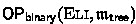is PSpace-hard.

### Proof (sketch)

Let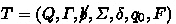be a deterministic Turing machine, where Q is the set of states, $$\varGamma$$ the tape alphabet,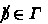the blank symbol, $$\varSigma \subseteq \varGamma$$ the input alphabet, $$\delta :Q\times \varGamma \not \rightarrow Q\times \varGamma \times \{-1,0,+1\}$$ the partial transition function, $$q_0$$ the initial state, and $$F\subseteq Q$$ the accepting states. We assume that T is polynomially space bounded, that is, there is a polynomial p such that on input words $$w\in \varSigma ^*$$, T only accesses the first $$p(|w|)$$ cells of the tape. For a word w, we denote by w[i] its ith letter. For some fixed word w, we construct a theory $$\mathcal {T}$$ using the following names, where $$k=p(|w|)$$:

• $$\mathsf {Start}$$ marks the inital and $$\mathsf {Accept}$$ an accepting configuration;

• to denote that we are in state $$q\in Q$$, we use a concept name $$S_q$$;

• for every $$a\in \varGamma$$ and $$i\in \{0,\ldots ,k\}$$, we use a concept name $$A_i^a$$ denoting that the letter a is on tape position i;

• for every $$i\in \{0,\ldots ,k\}$$, we use the concept name $$P_i^{+}$$ to denote that the head is currently on position i, and $$P_i^{-}$$ to denote that it is not;

• the role r is used to express the transitions between the configurations.

For convenience, we present the theory not in the required normal form, but aggregate conjunctions on the right. The following sentence describes the initial configuration.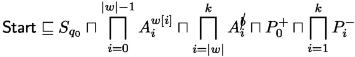(9)

The transition from one configuration to the next is encoded with the following sentences for every $$i\in \{0,\dots ,k\}$$ and every $$(q,a)\in Q\times \varGamma$$ with $$\delta (q,a)=(q',b,d)$$:

\begin{aligned} S_q\sqcap A_i^a\sqcap P_i^+&\sqsubseteq \exists r.S_{q'}\sqcap \forall r.A_i^b\sqcap \forall r.P_{i+d}^+\sqcap \sqcap _{j\in \{0,\ldots ,k\}\setminus \{i+d\}}\forall r.P_j^- \end{aligned}
(10)
\begin{aligned} A_i^a\sqcap P_i^-&\sqsubseteq \forall r.A_i^a \end{aligned}
(11)

Finally, we use the following sentences to detect accepting configurations and propagate the information of acceptance back to the initial configuration

\begin{aligned} S_f&\sqsubseteq \mathsf {Accept} \text { for all } f\in F, \end{aligned}
(12)
\begin{aligned} \mathsf {Accept}&\sqsubseteq \forall r^-.\mathsf {Accept} \end{aligned}
(13)

One can find a number q exponential in k and the size of T s.t. that there is a proof for $$\mathcal {T} \models \mathsf {Start} \sqsubseteq \mathsf {Accept}$$ with tree size at most q iff T accepts w. Using Proposition 13, we can extend $$\mathcal {T}$$ to a theory $$\mathcal {T} '$$ s.t. $$\mathcal {T} '\models \mathsf {Start} \sqsubseteq \mathsf {Accept}$$, while a proof of tree size q exists iff T accepts w (observe that $$\mathfrak {m} _{\mathsf {tree}} (\mathcal {P})\ge \mathfrak {m} _{\mathsf {depth}} (\mathcal {P})$$ holds for all proofs $$\mathcal {P}$$).   $$\square$$

## 5 Conclusion

We have investigated the complexity of finding optimal proofs w.r.t. quality measures that satisfy the property of being monotone recursive. Two important examples of this class of measures, depth and tree size, have been considered in detail in combination with exponential and polynomial derivers. The obtained results are promising: given a deriver, the search for an optimal proof for an entailment can be easier than producing all of the proofs by this deriver. The algorithms used to show the upper bounds can serve as building blocks for finding an optimal proof w.r.t. to a monotone recursive measure automatically.

We conjecture that weighted versions of tree size and depth, where sentences or inference steps can have associated rational weights, are also monotone recursive, and the generic upper bounds established in this paper can be straightforwardly applied to them. However, a more thorough study is required here, since the complexity of the decision problem depends on the exact way in which the weights are employed. This step towards weighted measures is motivated by user studies [1, 15, 24], demonstrating that different types of sentences and logical inferences can be more or less difficult to understand.